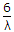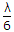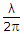# Electronics and Communication Engineering - Electromagnetic Field Theory

31.

Microwave signals follow the curvature of the earth and the phenomenon is known as

 A. tropospheric scatter B. duct propagation C. Faraday effect D. none of the above

Answer: Option B

Explanation:

No answer description available for this question. Let us discuss.

32.

In a carbon-zinc cell the function of the depolarizer is that

 A. it synthesizes the decomposed electrolyte B. it converts the produced hydrogen into water C. it absorbs the oxygen produced in the cell D. it prevents the fast chemical action on the zinc container

Answer: Option B

Explanation:

No answer description available for this question. Let us discuss.

33.

Induction and radiation fields are equal at a distance equal to

 A.B. 6λ C.D.Answer: Option D

Explanation:

No answer description available for this question. Let us discuss.

34.

The effective height of an antenna is slightly greater than physical height because

 A. wave velocity in conductor is less than its velocity in free space B. wave velocity in conductor is more than its velocity in free space C. resistance of conductor is less than that of free space D. none of the above

Answer: Option A

Explanation:

No answer description available for this question. Let us discuss.

35.

A long periodic antenna is a

 A. frequency independent antenna B. frequency dependent antenna C. directional antenna D. none of the above

Answer: Option A

Explanation:

No answer description available for this question. Let us discuss.

#### Current Affairs 2021

Interview Questions and Answers